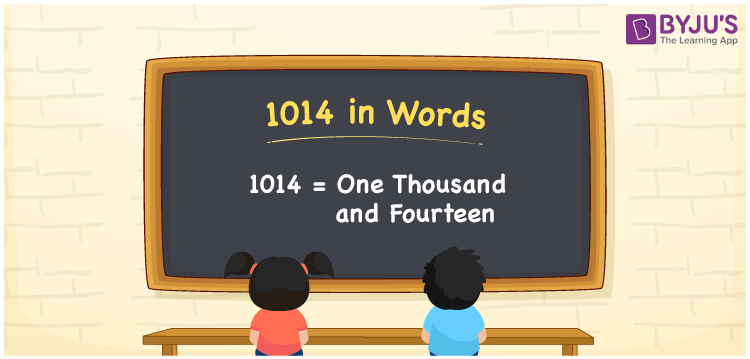# 1014 in Words

1014 in words is written as One thousand fourteen. In the International System of Numerals and the Indian System of Numerals, 1014 is written as One thousand fourteen. The number 1014 is a Cardinal Number as it could represent some quantity. For example, “this rope is about 1014 meters long”.

 1014 in Words One thousand fourteen One thousand fourteen in Number 1014

## 1014 in English Words

1014 in English words is read as “One thousand fourteen”.## How to Write 1014 in Words?

To write 1014 in words, we shall use the place value chart. In the place value chart, put 1 in the thousands, 0 in the hundreds, 1 in the tens, and 4 in the ones, respectively. Let us make a place value chart to write the number 1014 in words.

 Thousands Hundreds Tens Ones 1 0 1 4

Thus, we can write the expanded form as

1 × Thousand + 0 × Hundred + 1 × Ten + 4 × One

= 1 × 1000 + 0 × 100 + 1 × 10 + 4 × 1

= 1000 + 0 + 10 + 4

= 1014

= One thousand fourteen.

1014 is a natural number, the successor of 1013 and the predecessor of 1015.

1014 in words – One thousand fourteen

• Is 1014 an odd number? – No
• Is 1014 an even number? – Yes
• Is 1014 a perfect square number? – No
• Is 1014 a perfect cube number? – No
• Is 1014 a prime number? – No
• Is 1014 a composite number? – Yes

## Frequently Asked Questions on 1014 in Words

Q1

### How to write 1014 in words?

1014 in words is written as One thousand fourteen.
Q2

### How to write 1014 in the International and Indian System of Numerals?

In both, the system of numerals, 1014 in words, is written as One thousand fourteen.
Q3

### How to write 1014 in a place value chart?

In the place value chart, write 1 in the thousands, 0 in the hundreds, 1 in the tens, and 4 in the ones, respectively.